## RS Aggarwal Class 8 Solutions Chapter 3 Squares and Square Roots Ex 3D

These Solutions are part of RS Aggarwal Solutions Class 8. Here we have given RS Aggarwal Solutions Class 8 Chapter 3 Squares and Square Roots Ex 3D.

Other Exercises

Find the square root of each of the following numbers by using the method of prime factorization:

Question 1.
Solution:
225 = 3 x 3 x 5 x 5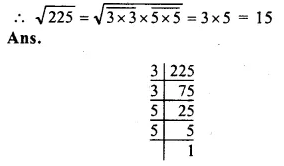Question 2.
Solution:
441 = 3 x 3 x 7 x 7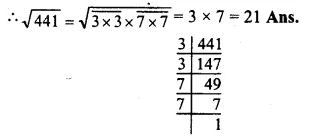Question 3.
Solution:
729 =Question 4.
Solution:
1296 = 2 x 2 x 2 x 2 x 3 x 3 x 3 x 3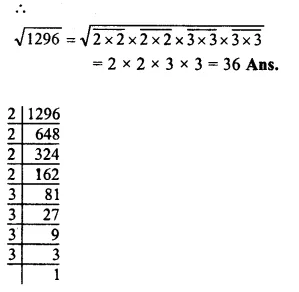Question 5.
Solution:
2025 =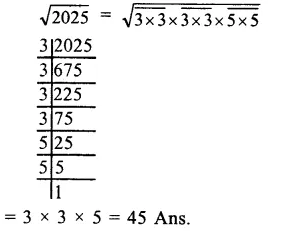Question 6.
Solution:
4096 =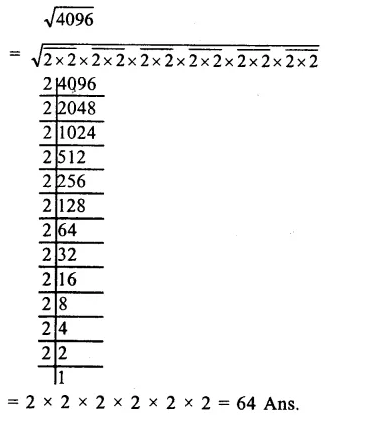Question 7.
Solution:
7056 = 2 x 2 x 2 x 2 x 3 x 3 x 7 x 7Question 8.
Solution:
8100 =Question 9.
Solution:
9216 =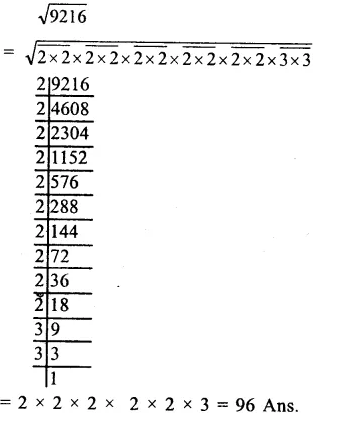Question 10.
Solution:
11025 =Question 11.
Solution:
15876 =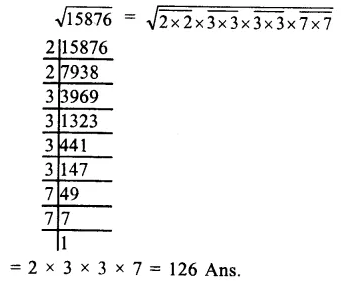Question 12.
Solution:
17424 =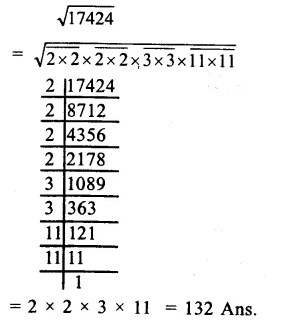Question 13.
Solution:
Factorizing 252, we get :
252 = $$\overline { 2X2 } X\overline { 3X3 } X7$$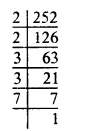To make it is a perfect square it must by multiplied by 7.
Square root of 252 x 7 = 1764
= 2 x 3 x 7 = 42 Ans.

Question 14.
Solution:
Factorizing 2925, we get :
2925 = $$\overline { 3X3 } X\overline { 5X5 } X13$$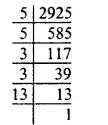To make it a perfect square it must be divided by 13.
2925 ÷ 13 = 225
Square root of 225 = 3 x 5 = 15 Ans.

Question 15.
Solution:
Let no. of rows = x
Then the number of plants in each row = x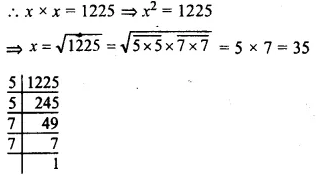Hence no. of rows = 35
and no. of plants in each row = 35

Question 16.
Solution:
Let no. of students in the class = x
Then contribution of each student = x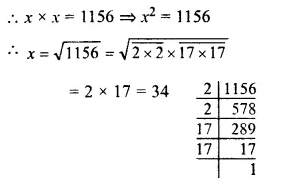No. of students of the class = 34 Ans.

Question 17.
Solution:
L.C.M. of 6, 9, 15 and 20
= 2 x 3 x 5 x 2 x 3 = 180
180 = $$\overline { 2X2 } X\overline { 3X3 } X5$$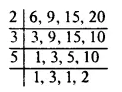To make it a perfect square, it must be multiplied by 5
Product = 180 x 5 = 900
Hence, the least square number = 900 Ans.

Question 18.
Solution:
L.C.M. of 8, 12, 15, 20 = 2 x 2 x 2 x 3 x 5= 120
120 = $$\overline { 2X2 }$$X2X3X5
To make it a perfect square it must be multiplied by 2 x 3 x 5 = 30
Then least perfect square = 120 x 30 = 3600Hope given RS Aggarwal Solutions Class 8 Chapter 3 Squares and Square Roots Ex 3D are helpful to complete your math homework.

If you have any doubts, please comment below. Learn Insta try to provide online math tutoring for you.